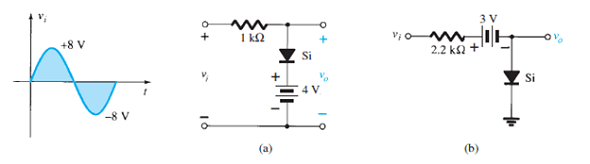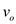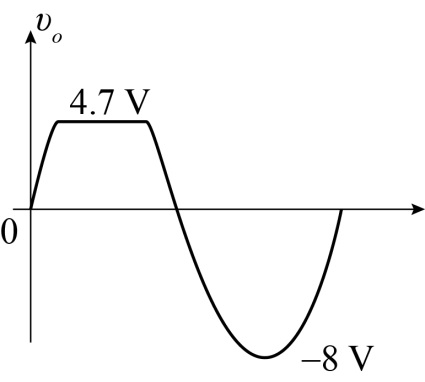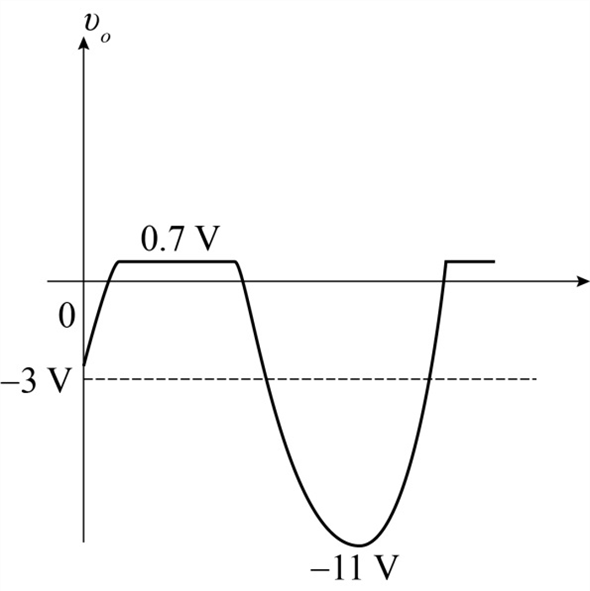Problem

# Determine vo for each network of Fig. 2.179 for the input shown.FIG. 2.179

Determine vo for each network of Fig. 2.179 for the input shown.

FIG. 2.179#### Step-by-Step Solution

Solution 1

(a)

Refer to the circuit diagram shown in Fig. $$2.176$$ (a) in the textbook.

As per Fig. $$2.176$$ (a), the Silicon Diode (Si) is ON when the input voltage $$v_{i} \geq 4.7 \mathrm{~V}$$.

For the condition $$v_{i}>4.7 \mathrm{~V}$$ the output voltage $$v_{o}$$ is given as:

$$v_{o}=V+V_{D}$$

Here,

Voltage $$V$$ is $$4.7 \mathrm{~V}$$

Silicon diode voltage $$V_{D}$$ is $$0.7 \mathrm{~V}$$

Substitute the corresponding values to obtain $$v_{o}$$ as follows:

\begin{aligned} v_{o} &=V+V_{D} \\ &=4 \mathrm{~V}+0.7 \mathrm{~V} \\ &=4.7 \mathrm{~V} \end{aligned}

Hence, the positive peak output voltage $$v_{o}$$ is $$4.7 \mathrm{~V}$$.

For the condition $$v_{i}<4.7 \mathrm{~V}$$ the diode is OFF and the output voltage $$v_{o}$$ is given as:

\begin{aligned} v_{o} &=v_{i} \\ &=-8 \mathrm{~V} \end{aligned}

Hence, the negative peak output voltage $$v_{o}$$ is $$-8 \mathrm{~V}$$.

The output voltage waveformis given as:Figure 1

(b)

Refer to the circuit diagram shown in Fig. 2.176 (b) in the textbook.

As per Fig. $$2.176$$ (b), the diode is ON when the input voltage $$v_{i} \geq 3.7 \mathrm{~V}$$.

So the output voltage $$v_{o}$$ is given as:

$$v_{o}=0.7 \mathrm{~V}$$

Hence, the positive output voltage $$v_{o}$$ is $$0.7 \mathrm{~V}$$.

As per Fig. $$2.176$$ (b), the diode is ON when the input voltage $$v_{i}<3.7 \mathrm{~V}$$. The diode current is same as that of the reverse biased current $$I_{D}=I_{R}=0 \mathrm{~mA}$$. Now the voltage across the resistor $$2.2 \mathrm{k} \Omega$$ is given as:

\begin{aligned} V_{2.2 \mathrm{k} \Omega} &=I R \\ &=(0 \mathrm{~mA}) R \\ &=0 \mathrm{~V} \end{aligned}

Now the output voltage $$v_{o}$$ is given as:

$$v_{o}=v_{i}-3 \mathrm{~V}$$

At the input voltage $$v_{i}=0 \mathrm{~V}$$ the output voltage $$v_{o}$$ is given as:

$$v_{o}=-3 \mathrm{~V}$$

Hence, the negative output voltage $$v_{o}$$ is $$-3 \mathrm{~V}$$.

Now when the input voltage $$v_{i}=-8 \mathrm{~V}$$ the output voltage $$v_{o}$$ is given as:

\begin{aligned} v_{o} &=-8 \mathrm{~V}-3 \mathrm{~V} \\ &=-11 \mathrm{~V} \end{aligned}

Hence, the negative output voltage $$v_{o}$$ is $$-11 \mathrm{~V}$$.

The output voltage waveformis given as:Figure 4